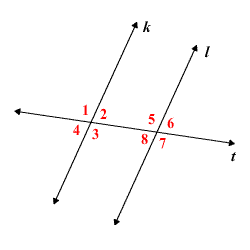# Alternate Exterior Angles

The term alternate exterior angles is often used when two lines are cut by a third line, a transversal .In the figure above, line $t$ is a transversal cutting lines $k$ and $l$ , and there are two pairs of alternate exterior angles:

$\angle 1$ and $\angle 7$

$\angle 4$ and $\angle 6$

The Alternate Exterior Angles Theorem states that if $k$ and $l$ are parallel , then the pairs of alternate exterior angles are congruent . That is,

$\angle 1\cong \angle 7$ and $\angle 4\cong \angle 6$ .

The converse of this theorem is also true.